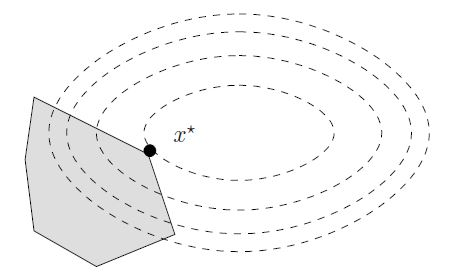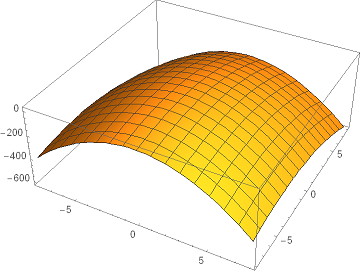# Quadratic programming

(diff) ← Older revision | Latest revision (diff) | Newer revision → (diff)
Jump to navigation Jump to search

This web page is a duplicate of https://optimization.mccormick.northwestern.edu/index.php/Quadratic_programming

Author: Jack Heider (ChE 345 Spring 2015)
Steward: Dajun Yue, Fengqi You

# IntroductionOptimizating of a quadratic function.12

Quadratic programming (QP) is the problem of optimizing a quadratic objective function and is one of the simplests form of non-linear programming.1 The objective function can contain bilinear or up to second order polynomial terms,2 and the constraints are linear and can be both equalities and inequalities. QP is widely used in image and signal processing, to optimize financial portfolios, to perform the least-squares method of regression, to control scheduling in chemical plants, and in sequential quadratic programming, a technique for solving more complex non-linear programming problems.3,4 The problem was first explored in the early 1950s, most notably by Princeton University's Wolfe and Frank, who developed its theoretical background,1 and by Harry Markowitz, who applied it to portfolio optimization, a subfield of finance.

# Problem formulation

A general quadratic programming formulation contains a quadratic objective function and linear equality and inequality constraints:2,5,6

${\text{min}}f(x)=q^{T}x+{\frac {1}{2}}x^{T}Qx$$s.t.Ax=a$$Bx\leq b$$x\geq 0$The objective function is arranged such that the vector $q$contains all of the (singly-differentiated) linear terms and $Q$contains all of the (twice-differentiated) quadratic terms. Put more simply, $Q$is the Hessian matrix of the objective function and $q$is its gradient. By convention, any constants contained in the objective function are left out of the general formulation.6 The one-half in front of the quadratic term is included to remove the coefficient (2) that results from taking the derivative of a second-order polynomial.

As for the constraints, the matrix equation $Ax=a$contains all of the linear equality constraints, and $Bx\leq b$are the linear inequality constraints.

When there are only inequality constraints ($Bx\leq b$), the Lagrangean is:6
$L(x,\lambda ,\mu )=q^{T}x+{\frac {1}{2}}x^{T}Qx+{\lambda }^{T}(Ax-a)+{\mu }^{T}(Bx-b)=0$## Global solutions

If the objective function is convex, then any local minimum found is also the sole global minimum. To analyze the function’s convexity, one can compute its Hessian matrix and verify that all eigenvalues are positive, or, equivalently, one can verify that the matrix Q is positive definite.6 This is a sufficient condition, meaning that it is not required to be true in order for a local minimum to be the unique global minimum, but will guarantee this property holds if true.

## KKT conditions

Solutions can be tested for optimality using Karush-Kuhn-Tucker conditions just as is done for other nonlinear problems:5

Condition 1: sum of gradients is zero:
$\triangledown L({x}^{*},{\lambda }^{*},{\mu }^{*})=q^{T}+{\frac {1}{2}}x^{T}Q+{\lambda }^{*T}A+{\mu }^{*T}B$Condition 2: all constraints satisfied:
$Ax^{*}-a=0$$Bx^{*}-b\leq 0$Condition 3: complementary conditions:
${\mu }^{T}Bx-b=0$${x}^{T},{\lambda }^{T},{\mu }^{T}\geq 0$## Solution strategies

Because quadratic programming problems are a simple form of nonlinear problem, they can be solved in the same manner as other non-linear programming problems. An unconstrained quadratic programming problem is most straightforward to solve: simply set the derivative (gradient) of the objective function equal to zero and solve.7 More practical (constrained) formulations are more difficult to solve. The typical solution technique when the objective function is strictly convex and there are only equality constraints is the conjugate gradient method. If there are inequality constraints ($Bx\leq b$), then the interior point and active set methods are the preferred solution methods. When there is a range on the allowable values of $x$(in the form $x^{L}\leq x\leq x^{U}$, which is the case for image and signal processing applications, trust-region methods are most frequently used.4 For all convex cases, an NLP solver in the optimization utility GAMS, such as KNITRO, MINOS, or CONOPT, can find solutions for quadratic programming problems.

# Numerical examplePlot of the unconstrained objective function. Figure generated using Wolfram Mathematica.

This example demonstrates how to determine the KKT point of a specific QP problem:

${\text{min}}$$f(x)=3{x_{1}}^{2}+{x_{2}}^{2}+2{x_{1}}{x_{2}}+x_{1}+6{x_{2}}+2$${\text{s.t.}}$$2{x_{1}}+3{x_{2}}\geq 4$${x_{1}},{x_{2}}\geq 0$Rewrite the constraints.
$g_{1}=-2{x_{1}}-3{x_{2}}+4\leq 0$$g_{2}=-{x_{1}}\leq 0$$g_{3}=-{x_{2}}\leq 0$Construct the gradients.
$\triangledown f={\begin{bmatrix}6{x_{1}}+2{x_{2}}+1\\2{x_{1}}+2{x_{2}}+6\end{bmatrix}},\triangledown {g_{1}}={\begin{bmatrix}-2\\-3\end{bmatrix}},\triangledown {g_{2}}={\begin{bmatrix}-1\\0\end{bmatrix}},\triangledown {g_{3}}={\begin{bmatrix}0\\-1\end{bmatrix}}$Assuming all constraints are satisfied, set the gradient equal to zero to attempt to find an optima.
$6{x_{1}}+2{x_{2}}+1=0$$2{x_{1}}+2{x_{2}}+6=0$${x_{1}}={\frac {5}{4}}$, ${x_{2}}=-{\frac {17}{4}}$Make constraints $g_{1}$and $g_{3}$, which are violated, active. Set both equal to zero.
${g_{1}}=-2{x_{1}}-3{x_{2}}+4=0$${g_{3}}=-{x_{2}}=0$Solve the resulting system of equations.
$\triangledown f+{\mu _{1}}\triangledown {g_{1}}+{\mu _{3}}\triangledown {g_{3}}=0$${g_{1}}=0$${g_{3}}=0$${\begin{bmatrix}6{x_{1}}+2{x_{2}}+1\\2{x_{1}}+2{x_{2}}+6\end{bmatrix}}+{\mu _{1}}{\begin{bmatrix}-2\\-3\end{bmatrix}}+{\mu _{3}}{\begin{bmatrix}0\\-1\end{bmatrix}}$$-2{x_{1}}-3{x_{2}}+4=0$$-{x_{2}}=0$Formulating the system as one matrix and row reducing is one of the simplest ways to solve. Doing so yields:
${x_{1}}=2,{x_{2}}=0,{\mu _{1}}=6.5,{\mu _{3}}=9.5$Drop constraint $g_{3}$because ${\mu _{3}}$is negative and resolve the system. Doing so yields:
${x_{1}}=0.5,{x_{2}}=1,{\mu _{1}}=3$Which yields an objective function value of $f=11.25$Verify linear dependence of the gradient:
$\triangledown f+{\mu _{1}}\triangledown {g_{1}}$${\begin{bmatrix}6\\9\end{bmatrix}}+3{\begin{bmatrix}-2\\-3\end{bmatrix}}={\begin{bmatrix}0\\0\end{bmatrix}}\therefore$Verify the uniqueness of the solution:
${\triangledown }^{2}f(x)={\begin{bmatrix}6&2\\2&1\end{bmatrix}}\rightarrow {\begin{bmatrix}6-\lambda &2\\2&1-\lambda \end{bmatrix}}=0\rightarrow \lambda =0.298,6.702$Because both eigenvalues are positive, the Hessian matrix is positive determinant, and this local minimum is the global minimum of the objective function given these constraints.

# Applications

A few of the many quadratic programming applications are discussed in more detail and accompanied with general models below, and a list of other areas in which QP is important is presented as well.

• Quadratic knapsack problem (QKP)

In the standard knapsack problem, there are a number of items with different weights and values, and the items are selected based on which combination yields the highest overall value without exceeding the overall weight limit of the knapsack.

In the quadratic knapsack problem, the objective function is quadratic or, more specifically, bilinear, and the constraints are the same as in the typical knapsack problem.8 QKP's are used in designing email servers and to optimize the locations of "nodes" in applications such as positioning transportation hubs like airports and train stations.8 Additionally, the problem can model situations in which a limited number of people are assigned to complete specific objectives that require them to interact.9 One formulation is presented below:8
${\text{max or min}}$$x^{T}Qx$$Ax\leq a$$x\in {B}^{n}$The quadratic knapsack problem, although it looks very simple, is NP-hard and is thus difficult to solve. To make obtaining solutions easier, these problems are often linearized.8

• Model predictive control

Quadratic programming also has important applications in chemical engineering. Model predictive control (MPC) is a group of algorithms that help manage production in chemical plants by dictating production in each batch. While often formulated as linear programs because the resulting models are more stable, robust and easier to solve, MPC models are sometimes made with quadratic programming.11 As an example of its utility, quadratic programming was used by Di Ruscio in an MPC algorithm for a thermomechanical pulping process, which a method for making paper.11

• Least squares

Least squares regression is one of the most common types of regression, and works by minimizing the sum of the squares of the difference between data points and a proposed fit. Quadratic optimization is one method that can be used to perform a least squares regression and is more flexible than most linear methods. One formulation for a quadratic programming regression model is as follows:3

${\text{min}}$$e'Ie$$e=Y-X\beta$In this model, $\beta$and $e$are the unknown regression parameters, $I$is an identity matrix, and $X$and $Y$contain data about the independent and dependent variables respectively.3

• Other applications

Quadratic programming is used in a wide range of applications not touched upon in the sample presented above. Other major areas in which QP's are relied upon include signal and image processing12 and a subfield of optimization called partial differential constrained optimization.3 QP's are also extensively used in finance, as variance, which is used to measure risk, is a function containing squares.13,14,15 More specifically, Markowitz won the 1990 Nobel Prize in Economics for his widely-used model that employs quadratic programming to optimizes the amount of risk taken on based on variances.14

Additionally, Sequential quadratic programming, an algorithm for solving more complicated NLP's that uses QP subproblems, is one of the most important applications.

# Conclusion

Quadratic programming, the problem of optimizing a quadratic function, have been widely used since its development in the 1950s because it is a simple type of non-linear programming that can accurately model many real world systems, notably ones dependent on two variables. Problems formulated this way are straightforward to optimize when the objective function is convex. QP has applications in finance, various types of computer systems, statistics, chemical production, and in algorithms to solve more complex NLP's.